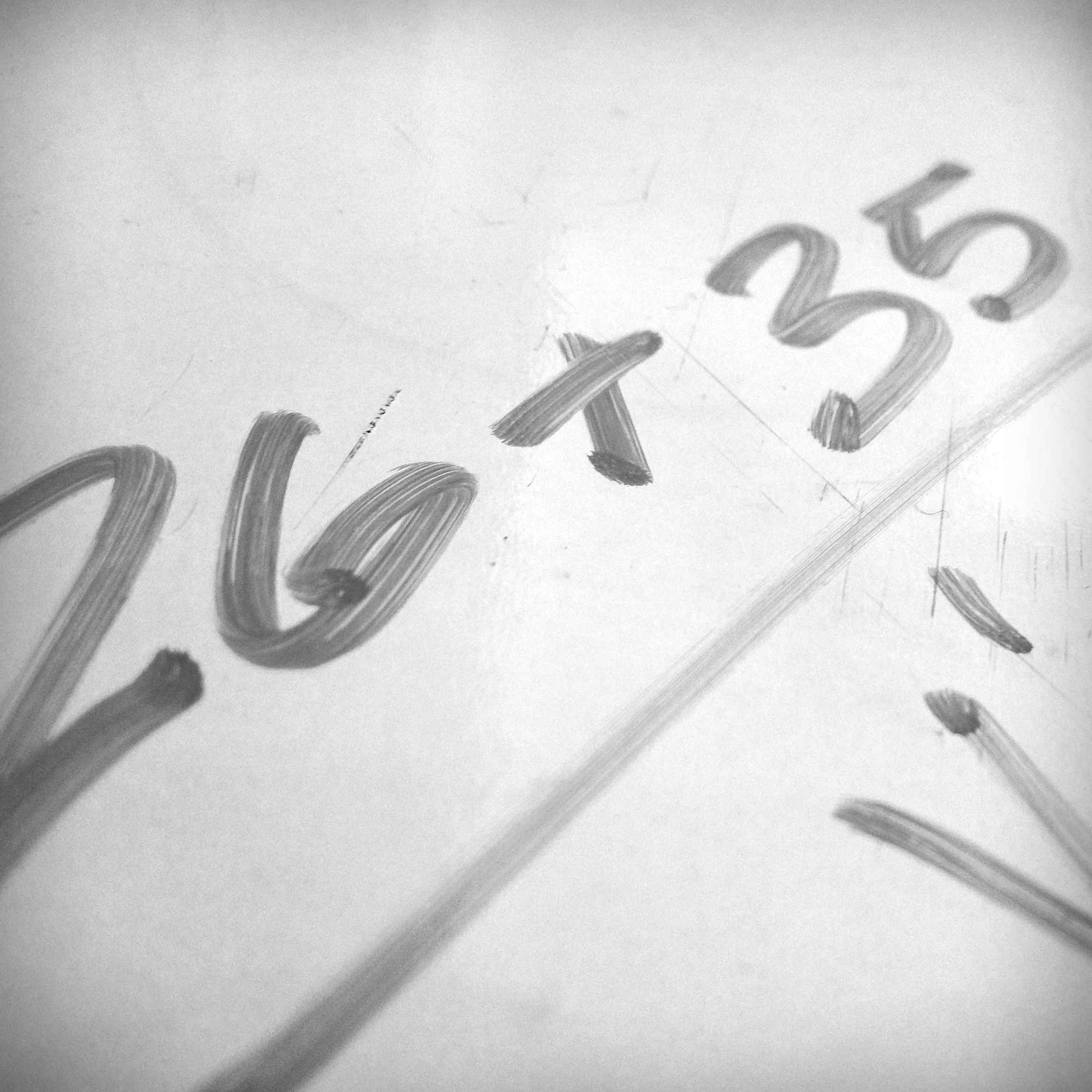Home » Attitude, Do The Math

# Attitude, Do The MathAs you start a new week this week consider the following question.

If you were to assign a point value on a scale of 0-100, with 100 being the best score, to the following attributes in life, which attribute would you give the highest score?

Hard Work
Knowledge
Luck
Attitude

If you’re having trouble coming up with a score, consider the math.

If
A B C D E F G H I J K L M N O P Q R S T U V W X Y Z
is equal to
1 2 3 4 5 6 7 8 9 0 10 11 12 13 14 15 16 17 18 19 20 21 22 23 24 25 26

Then

H+A+R+D+W+O+R+K =
8+1+18+4+23+15+18+11 = 98

K+N+O+W+L+E+D+G+E =
11+14+15+23+12+5+4+7+5 = 96

L+U+C+K =
12+21+3+11 = 47

A+T+T+I+T+U+D+E =
1+20+20+9+20+21+4+5 = 100

You can’t argue with the numbers. Of the four attributes listed, only one has a perfect score.  Give it some thought, and remember to shake hands with a smile!